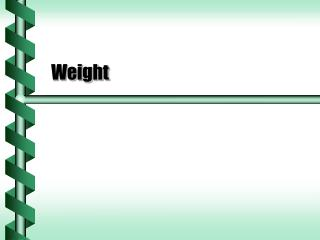DownloadDownload PresentationWeight

# Weight

Download Presentation## Weight

- - - - - - - - - - - - - - - - - - - - - - - - - - - E N D - - - - - - - - - - - - - - - - - - - - - - - - - - -
##### Presentation Transcript

1. Weight

2. Acceleration of Gravity • Objects that fall to the Earth all experience an acceleration. • The acceleration due to gravity is g = 9.8 m/s2. • This acceleration must be due to a force.

3. The acceleration of a falling mass m is -g. The force on the mass is found from F = ma (action). This gravitational force is F = -mg. Force of Gravity Kinematic view Dynamic view

4. Gravity at Rest • The force of gravity acts on all objects all the time. • If an object is at rest, the law of inertia says that the net force is zero. • There must be a force opposite to gravity that cancels it out. F = -Fgrav =mg Fgrav = -mg

5. Normal Force • The force that opposes gravity for objects on the ground is called the normal force. • It is perpendicular (normal) to the plane of the ground. • The force is a result of the law of reaction. FN = mg Fsb = -Fbs =mg

6. Normal Force and Weight • The normal force pushing up against gravity can be measured. • We measure weight with a scale that measures normal force. • Weight is a force, not a mass. • Pounds measure weight, so force (not mass) can be measured in pounds.

7. Accelerated Weight • An elevator is accelerating downward at 2.0 m/s2. • The person has a mass of 70 kg. • What weight is on the scale? • Add all the forces, but the net force is –ma = FN – mg. • Solve for FN = m (g – a) • The scale shows 56 kg.

8. Weightlessness • If the elevator accelerated downward at g, the normal force would become 0. • The person would feel weightless. • An object in free fall is weightless, but not massless. Microgravity research at NASA next